# I did a redox titration. I need help calculating the concentration of my KMnO4 solution. So i fir...

I did a redox titration. I need help calculating the concentration of my KMnO4 solution. So i first measured .2125 g of Na2C2O4 and put it into a flask. Next I transferred 250 ml of 1 M H2SO4 solution into the flask and dissolved the salt. I then titrated it with KMnO4 with 27.2 ml of KMnO4 solution. What is the concentration of my KMnO4 solution? Please show all the work.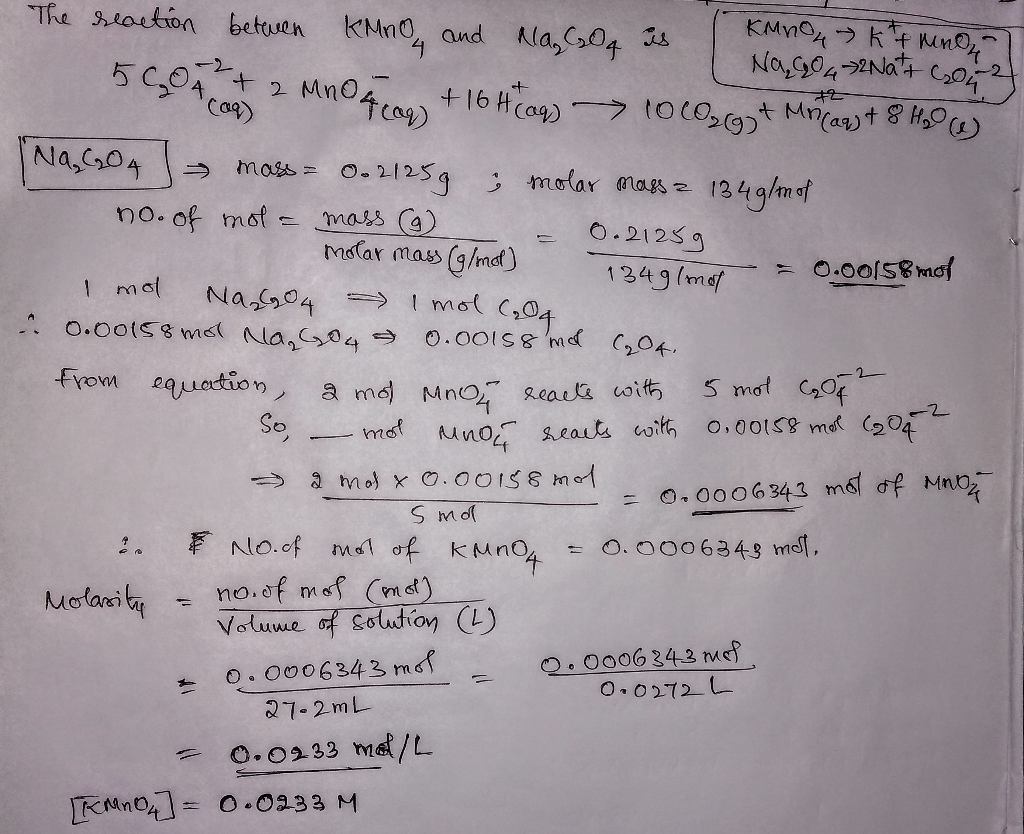thanks and hope you like the answer

##### Add Answer of: I did a redox titration. I need help calculating the concentration of my KMnO4 solution. So i fir...
Similar Homework Help Questions
• ### A titration is a procedure for determining the concentration of a solution by allowing it to...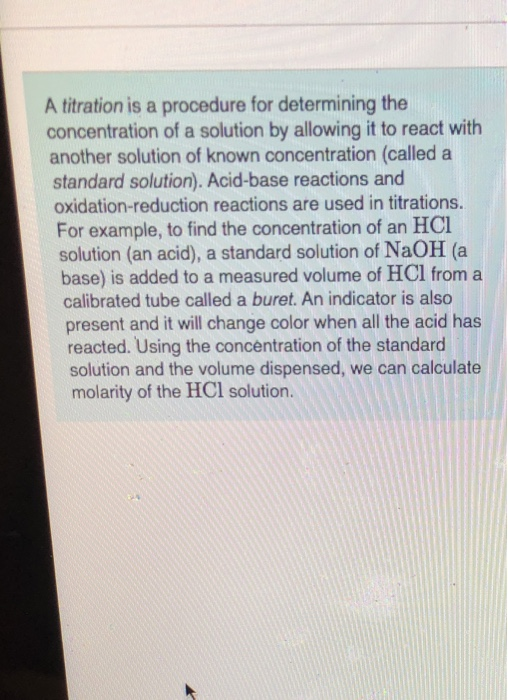A titration is a procedure for determining the concentration of a solution by allowing it to react with another solution of known concentration (called a standard solution). Acid-base reactions and oxidation-reduction reactions are used in titrations. For example, to find the concentration of an HCI solution (an acid), a standard solution of NaOH (a base) is added to a measured volume of HCl from a calibrated tube called a buret. An indicator is also present and it will change color...

• ### 1. (4p) In an acidic aqueous solution, Fe2+ ions are oxidized to Fe3+ ions by MnO4": 5Fe2+(aq) + MnO4 (aq) + 8H(aq) → 5...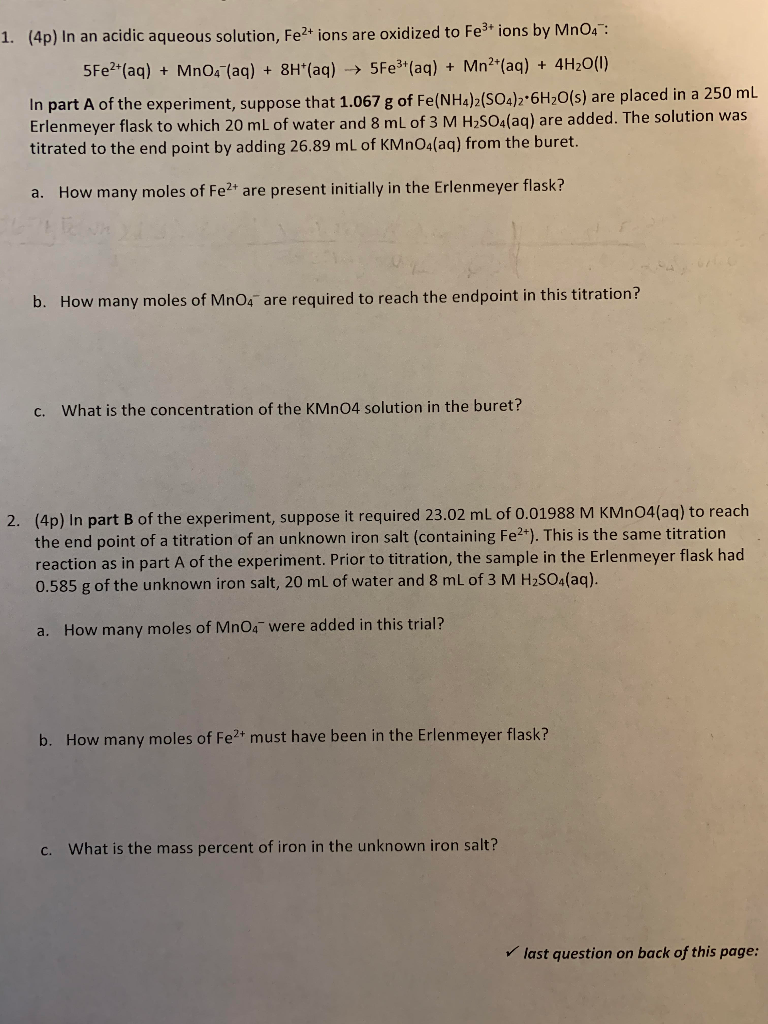1. (4p) In an acidic aqueous solution, Fe2+ ions are oxidized to Fe3+ ions by MnO4": 5Fe2+(aq) + MnO4 (aq) + 8H(aq) → 5Fe3+ (aq) + Mn2(aq) + 4H2O(1) In part A of the experiment, suppose that 1.067 g of Fe(NH4)2(SO4)2.6H2O(s) are placed in a 250 ml Erlenmeyer flask to which 20 mL of water and 8 mL of 3 M H2SO4(aq) are added. The solution was titrated to the end point by adding 26.89 mL of KMnO4(aq) from the...

• ### hydrogen peroxide titration, concentration of KMnO4 is 0.025 and the concentration of H2O2 from the mol...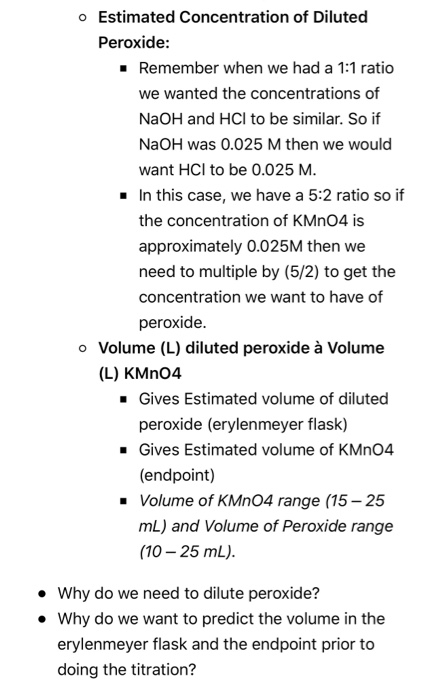hydrogen peroxide titration, concentration of KMnO4 is 0.025 and the concentration of H2O2 from the mol ratio is 0.882 M o Estimated Concentration of Diluted Peroxide: . Remember when we had a 1:1 ratio we wanted the concentrations of NaOH and HCl to be similar. So if NaOH was 0.025 M then we would want HCl to be 0.025 M. In this case, we have a 5:2 ratio so if the concentration of KMnO4 is approximately 0.025M then we need...

• ### TITRATION: I did the first page, but I need help with the rest; I'm lost. I...TITRATION: I did the first page, but I need help with the rest; I'm lost. I uploaded the first page because you need to reference is for the preceding questions. Pre-Lab Assignment: Titration (Week 11) Name: Group: Chapter 8 Section 7 in your lecture textbook covers the calculations needed for this week's lab. They are very similar to calculations we covered in Chapter 7. Complete the following before coming to lab. It will be GRADED! Given the balanced equation: HBraq)...

• ### need help with the Molarity of both parts EXPERIMENT 8: AN OXIDATION-REDUCTION TITRATION DATA SHEET 0.2M...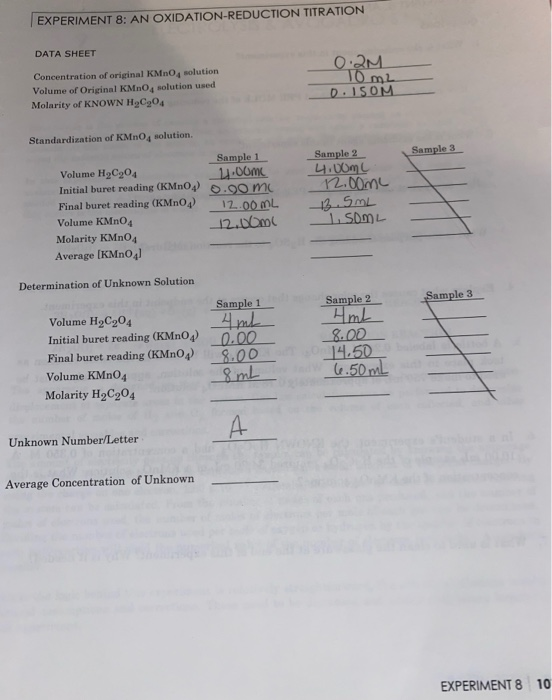need help with the Molarity of both parts EXPERIMENT 8: AN OXIDATION-REDUCTION TITRATION DATA SHEET 0.2M 10 m2 Concentration of original KMnO solution Volume of Original KMnO4 solution used Molarity of KNOWN HOCg04 D.ISOM Standardization of KMnO solution. Sample 3 Sample 1 Volume H2C204 11.00ML Initial buret reading (KMnO4) .00 mc Final buret reading (KMnO4) 12.00ML Volume KMnO4 12.00ml Molarity KMnO4 Average (KMnO4) Sample 2 4:00ML 12.00mL 13.5ML . SOL Determination of Unknown Solution Sample 3 Sample 1 4ml Volume...

• ### In the United States, table salt is “iodized” and contains NaCl and a small amount of KI. The iodide was added to help p...

In the United States, table salt is “iodized” and contains NaCl and a small amount of KI. The iodide was added to help prevent thyroid disorders in the population. The iodide concentration in table salt was measured using the following steps: 1.000 g of table salt was dissolved in 100.0 mL of water. The salt solution was titrated using 4.00 × 10−5 M Ag+ and a Volhard titration (back titration). 15.00 mL of 4.00 × 10−5 M Ag+ solution was...

• ### I need help with this question

I need help with this question!! a sample of pure KHP weighing .8097g dissolved in water and titrated with 40.25 mL of NaOH solution. The same NaOH solution was used to titrate a solution of a weak diprotic acid H2X. A sample of 0.18694g of the weak acid was first dissolved in water in a 100 mL volumetric flask creating a slution of 100.00 mL. A 25.00 mL portion of this solution was tuitrated with the sodium hydroxide solution. The...

• ### I need it right now, please help me?? PROCEDURE PART I: DILUTING THE VINEGAR SOLUTION The...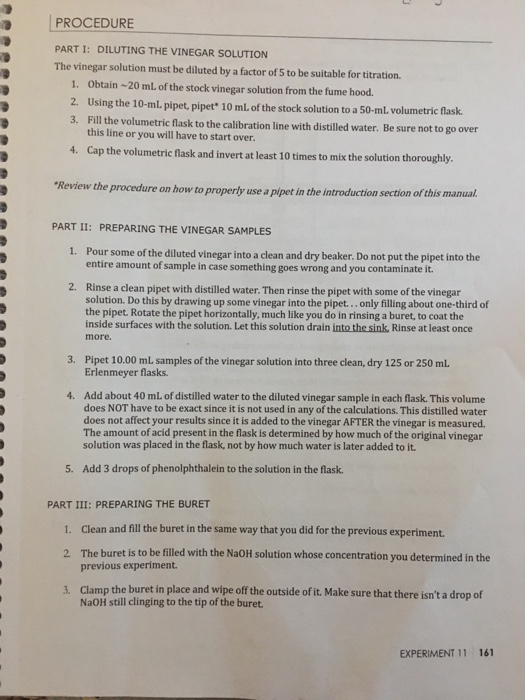I need it right now, please help me?? PROCEDURE PART I: DILUTING THE VINEGAR SOLUTION The vinegar solution must be diluted by a factor of 5 to be suitable for titration. 1. Obtain - 20 ml of the stock vinegar solution from the fume hood. 2. Using the 10-ml. pipet, pipet" 10 mL of the stock solution to a 50-ml volumetric flask. 3. Fill the volumetric flask to the calibration line with distilled water. Be sure not to go over...

• ### need help with part a and part b Calculating Mass Percent Concentration Review I Consta The...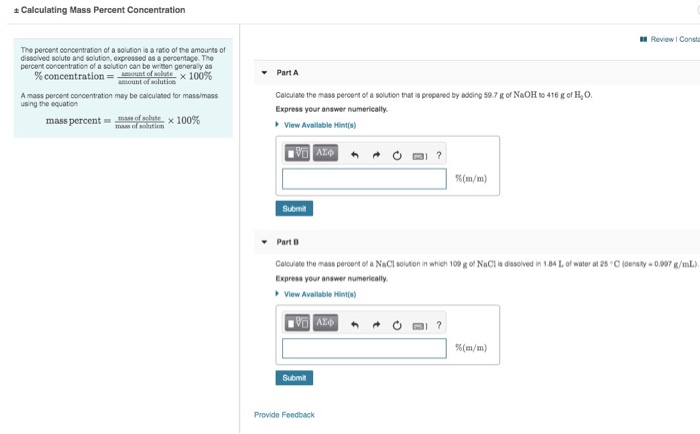need help with part a and part b Calculating Mass Percent Concentration Review I Consta The percent concentration of a solution is a ratio of the amounts of dissolved solute and solution, exprossed as a percentage. The percent concentration of a solution can be wrimen generaly as Ount of sobate Part A % concentration tof 100 % Calculate the mass percent of a solution that is prepared by adding 59.7 g of NaOH to 416 g of H,O. A mass...

• ### Need help with H please A student followed the procedure of this experimer 3. commercial bleaching...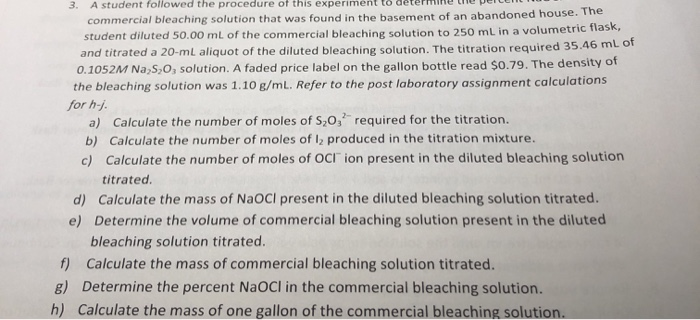Need help with H please A student followed the procedure of this experimer 3. commercial bleaching solution that was found in the basement of an abandoned house. The student diluted 50.00 mL of the commercial bleaching solution to 250 mL in a volumetric flask, and titrated a 20-mL aliquot of the diluted bleaching solution. The titration required 35.46 mL of 0.1052M NaS, O, solution. A faded price label on the gallon bottle read \$0.79. The density of the bleaching solution...

Need Online Homework Help?